Find solution of equations- Equations given

Chapter 4 Class 12 Determinants
Concept wise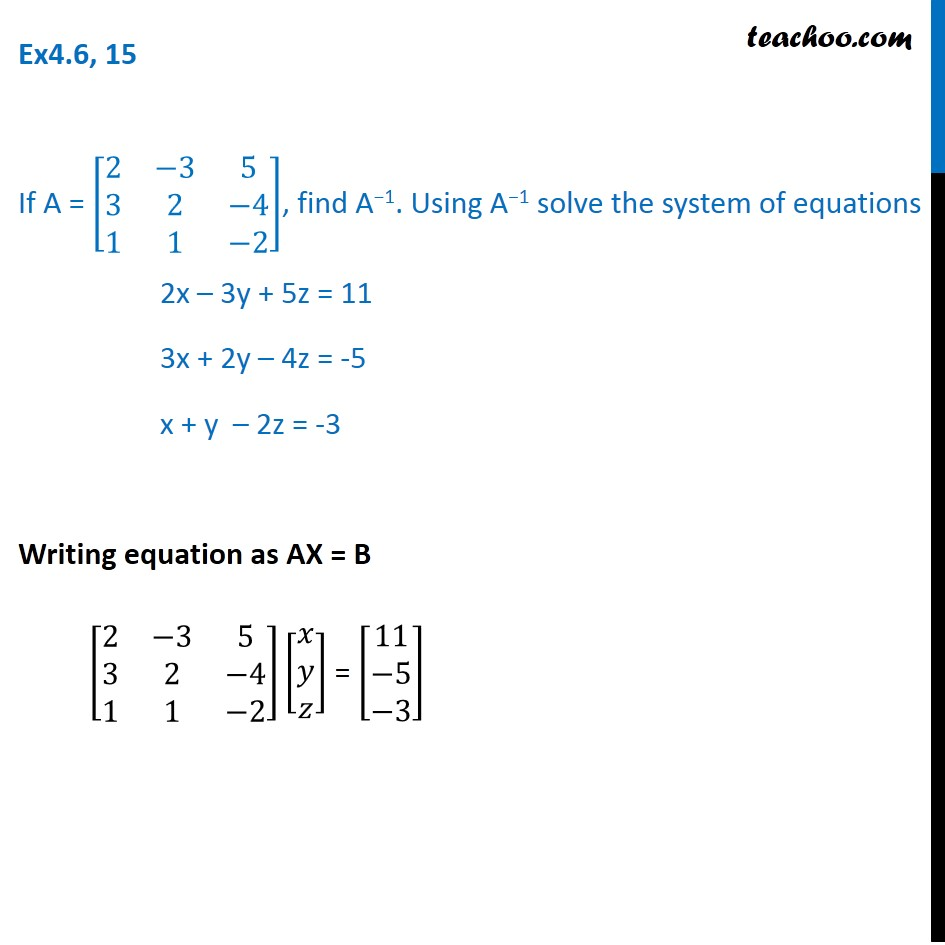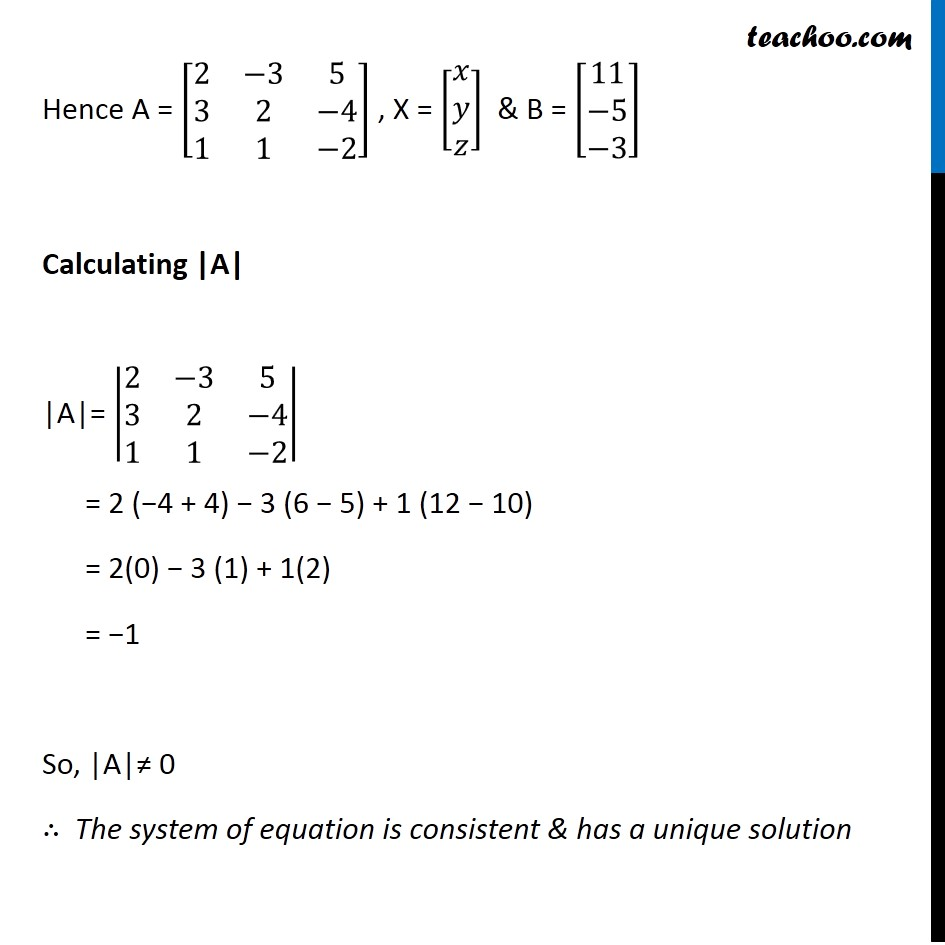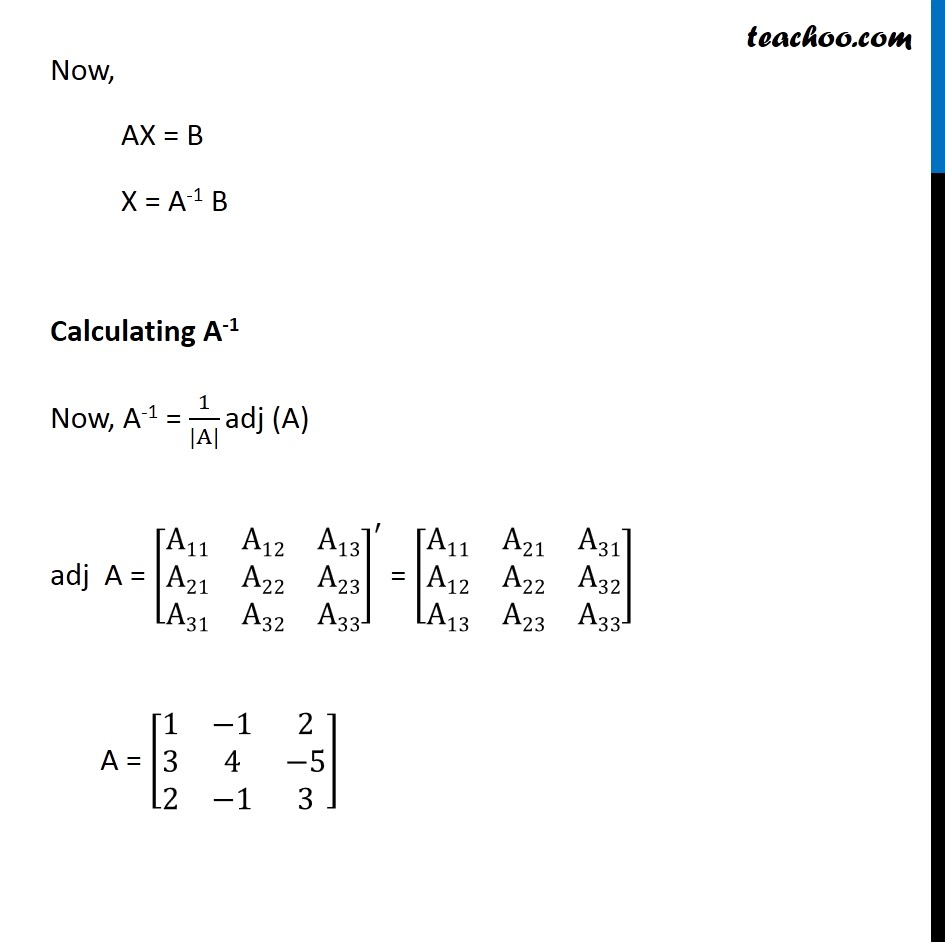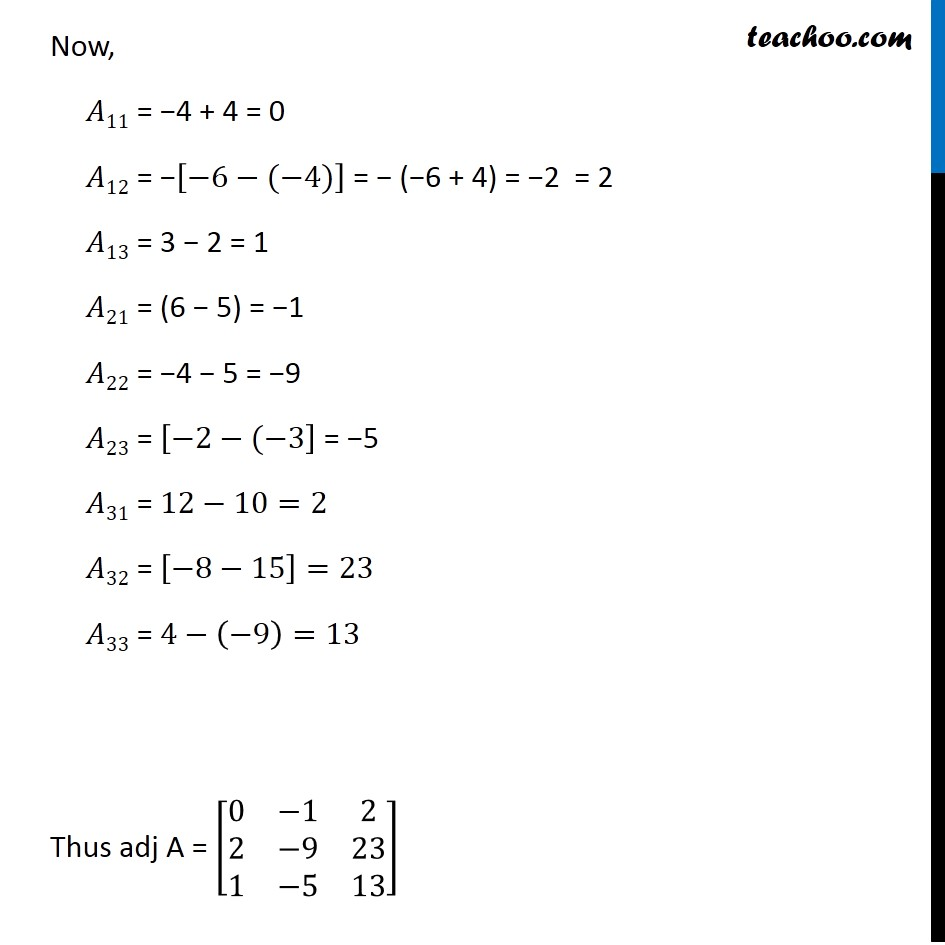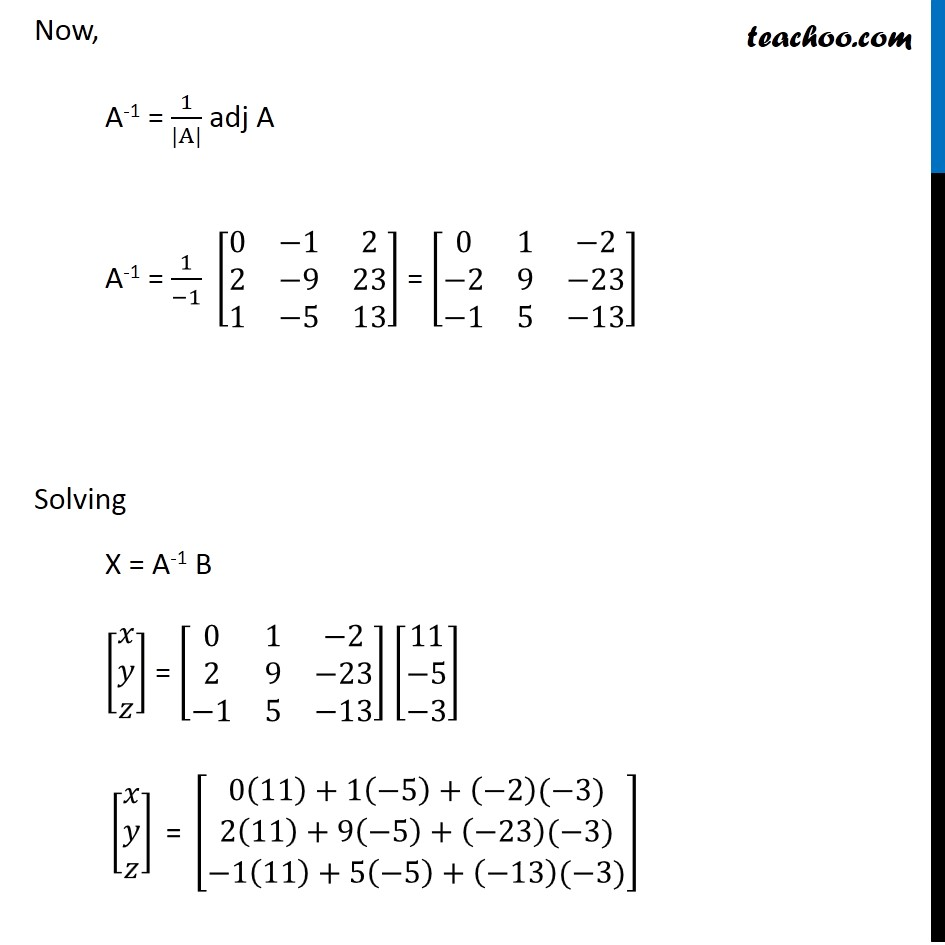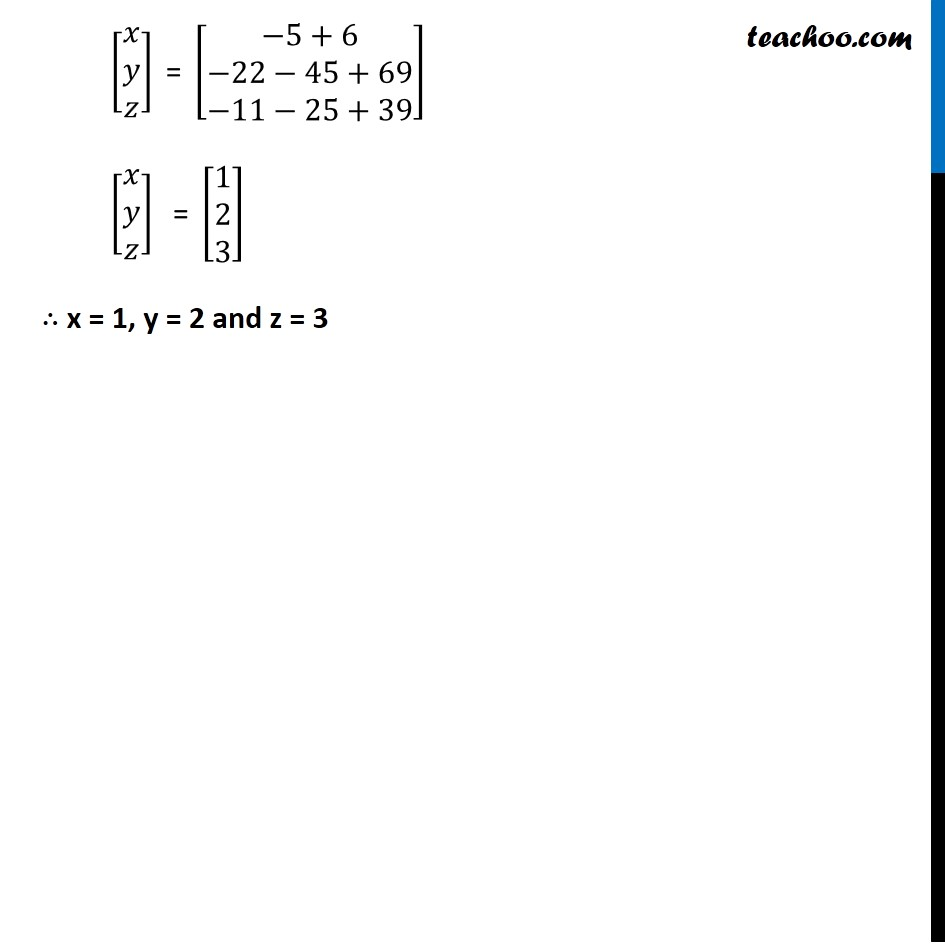Learn in your speed, with individual attention - Teachoo Maths 1-on-1 Class

### Transcript

Ex4.6, 15 If A = [■8(2&−3&[email protected]&2&−[email protected]&1&−2)], find A−1. Using A−1 solve the system of equations 2x – 3y + 5z = 11 3x + 2y – 4z = -5 x + y – 2z = -3 Writing equation as AX = B [■8(2&−3&[email protected]&2&−[email protected]&1&−2)][■8(𝑥@𝑦@𝑧)] = [■8(11@−5@−3)] Ex4.6, 15 If A = [■8(2&−3&[email protected]&2&−[email protected]&1&−2)], find A−1. Using A−1 solve the system of equations 2x – 3y + 5z = 11 3x + 2y – 4z = -5 x + y – 2z = -3 Writing equation as AX = B [■8(2&−3&[email protected]&2&−[email protected]&1&−2)][■8(𝑥@𝑦@𝑧)] = [■8(11@−5@−3)] Ex4.6, 15 If A = [■8(2&−3&[email protected]&2&−[email protected]&1&−2)], find A−1. Using A−1 solve the system of equations 2x – 3y + 5z = 11 3x + 2y – 4z = -5 x + y – 2z = -3 Writing equation as AX = B [■8(2&−3&[email protected]&2&−[email protected]&1&−2)][■8(𝑥@𝑦@𝑧)] = [■8(11@−5@−3)] Hence A = [■8(2&−3&[email protected]&2&−[email protected]&1&−2)] , X = [■8(𝑥@𝑦@𝑧)] & B = [■8(11@−5@−3)] Calculating |A| |A|= |■8(2&−3&[email protected]&2&−[email protected]&1&−2)| = 2 (−4 + 4) − 3 (6 − 5) + 1 (12 − 10) = 2(0) − 3 (1) + 1(2) = −1 So, |A|≠ 0 ∴ The system of equation is consistent & has a unique solution Now, AX = B X = A-1 B Calculating A-1 Now, A-1 = 1/(|A|) adj (A) adj A = [■8(A11&A12&[email protected]&A22&[email protected]&A32&A33)]^′ = [■8(A11&A21&[email protected]&A22&[email protected]&A23&A33)] A = [■8(1&−1&[email protected]&4&−[email protected]&−1&3)] Now, 𝐴11 = −4 + 4 = 0 𝐴12 = −[−6−(−4)] = − (−6 + 4) = −2 = 2 𝐴13 = 3 − 2 = 1 𝐴21 = (6 − 5) = −1 𝐴22 = −4 − 5 = −9 𝐴23 = [−2−(−3] = −5 𝐴31 = 12−10=2 𝐴32 = [−8−15]=23 𝐴33 = 4−(−9)=13 Thus adj A = [■8(0&−1&[email protected]&−9&[email protected]&−5&13)] Now, A-1 = 1/(|A|) adj A A-1 = 1/(−1) [■8(0&−1&[email protected]&−9&[email protected]&−5&13)] = [■8(0&1&−2@−2&9&−23@−1&5&−13)] Solving X = A-1 B [■8(𝑥@𝑦@𝑧)] = [■8(0&1&−[email protected]&9&−23@−1&5&−13)] [■8(11@−5@−3)] " " [■8(𝑥@𝑦@𝑧)]" = " [█(0(11)+1(−5)+(−2)(−3)@2(11)+9(−5)+(−23)(−3)@−1(11)+5(−5)+(−13)(−3))] " " [■8(𝑥@𝑦@𝑧)]" = " [█(−5+6@−22−45+69@−11−25+39)] " " [■8(𝑥@𝑦@𝑧)]" = " [■8([email protected]@3)] "∴ x = 1, y = 2 and z = 3 "